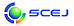## \$B9V1i%W%m%0%i%`!J%;%C%7%g%sJL!K(B

English page

### \$B0lHL9V1i(B

#### C104-C109, C113-C117, C202-C209, D104-D109, D113-D117

\$B:G=*99?7F|;~!'(B2014-11-19 12:59:10
\$B9V1i(B
\$B;~9o(B
\$B9V1i(B
\$BHV9f(B
\$B9V1iBjL\!?H/I=\$B%-!<%o!<%I(B\$BJ,N`(B
\$BHV9f(B
\$B\$BHV9f(B
C \$B2q>l!&Bh(B 1 \$BF|(B
(10:00\$B!A(B12:00) (\$B:BD9(B \$B?7A%(B \$B9,Fs!&0KF#(B \$BOB9((B)
10:00\$B!A(B 10:20C104\$B?)IJE`7k4%Ag%W%m%;%9\$N4J0W\$J?t3X%b%G%k(B
(\$B5~Bg1!G@(B) \$B!{(B(\$B@5(B)\$BCf@n(B \$B5fLi(B\$B!&(B (\$BE7Ln
drying
food
freeze-drying
4-h49
10:20\$B!A(B 10:40C105\$B5^=L>.5^3HBgN.O)Fb\$K\$*\$1\$k9bJ,;RMOM;BN\$NN.F02r@O(B - \$B%^%k%A%9%1!<%k%7%_%e%l!<%7%g%s\$K\$h\$k%"%W%m!<%A(B -
(\$B5~Bg1!9)(B) \$B!{(B(\$B3X(B)\$B86ED(B \$B9@J?(B\$B!&(B (\$B@5(B)\$BC+8}(B \$B5.;V(B
Multi-scale simulation
Particle method
12-l76
10:40\$B!A(B 11:00C106\$BG4CF@-N.BN\$rBP>]\$H\$7\$?@55U8r8_2sE>Mc\$N3IYBFC@-(B
(\$B?@8MBg9)(B) \$B!{IZKq(B \$BJ8EP(B\$B!&(B (\$B?@8MBg1!9)(B) (\$B@5(B)\$B8VED(B \$B1YG7(B\$B!&(B (\$B:eBg1!9)(B) (\$B@5(B)\$BJ?ED(B \$BM:;V(B\$B!&(B (\$B?@8MBg1!9)(B) (\$B@5(B)\$BNkLZ(B \$BMN(B\$B!&(B (\$B@5(B)\$BF|=P4V(B \$B\$k\$j(B
Non Newtonian
flow pattern
2-b59
11:00\$B!A(B 11:20C107(\$B9V1iCf;_(B)

100118
11:20\$B!A(B 11:40C108\$BJBNs%^%\$%/%m%W%m%;%9\$K\$*\$1\$k%*%j%U%#%9IUN.O)\$rMQ\$\$\$?N.BNJ,G[(B
(\$B5~Bg9)(B) \$B!{(B(\$B3X(B)\$BEmLn(B \$B9'9((B\$B!&(B (\$B@5(B)\$BEBB<(B \$B=\$(B\$B!&(B (\$B3X(B)\$B5\NS(B \$B7=Je(B\$B!&(B (\$B@5(B)\$BC+8}(B \$BCR(B\$B!&(B (\$B@5(B)\$BD9C+It(B \$B?-<#(B
microreactor
orifice
pressure drop reduction
5-f74
11:40\$B!A(B 12:00C109van der Waals\$BM}O@\$K=>\$&1UE)\$N%@%\$%J%_%/%9(B
(\$B5~Bg1!9)(B) \$B!{(B(\$B3X(B)\$BKL@n(B \$B??;K(B\$B!&(B (\$B@5(B)\$BC+8}(B \$B5.;V(B
van der Waals theory
vapor-liquid coexistence
evaporation
2-e75
(13:00\$B!A(B14:40) (\$B:BD9(B \$B5FCS(B \$B>f9,!&Cf@n(B \$B5fLi(B)
13:00\$B!A(B 13:20C113Effect of spray drying temperature on aggregated-casein structures and its digestibility.
(\$BJ<8K8)Bg1!9)(B) \$B!{(B(\$B3X(B)Jarunglumlert Teeraya\$B!&(B (\$B5~Bg1!G@(B) (\$B@5(B)\$BCf@n(B \$B5fLi(B\$B!&(B (\$B@5(B)\$B0BC#(B \$B=\$Fs(B
Spray drying
Aggregation
Casein
4-h60
13:20\$B!A(B 13:40C114\$B8GBN1v4p%+!<%\%s%J%N?(G^\$r;HMQ\$7\$?;iKC;@%a%A%k%(%9%F%k\$N9g@.(B
(\$B5~Bg1!9)(B) \$B!{(B(\$B3X(B)\$B;3ED(B \$B9WJ?(B\$B!&(B (\$B@5(B)\$B:4Ln(B \$B5*>4(B\$B!&(B (\$B@5(B)\$BEDLg(B \$BH%(B
carbon nanohorn
solid base catalyst
biodiesel
5-a19
13:40\$B!A(B 14:00C115\$B%^%\$%/%m%j%"%/%?!<\$H6bB0C4;}C:AG?(G^\$rMQ\$\$\$?%K%H%m%Y%s%<%s\$N?eAGE:2CH?1~(B
(\$B5~Bg9)(B) \$B!{(B(\$B3X(B)\$BK\B?(B \$BCN2B(B\$B!&(B (\$B@5(B)\$BJ!ED(B \$B5.;K(B\$B!&(B (\$B@5(B)\$BKR(B \$BBYJe(B\$B!&(B (\$B5~Bg4D2J%;(B) (\$B@5(B)\$BCf@n(B \$B9@9T(B\$B!&(B (\$B5~Bg9)(B) (\$B@5(B)\$BA0(B \$B0lW"(B
Microreactor
packed-bed reactor
carbon catalyst
5-f68
14:00\$B!A(B 14:20C116\$B:xBN=E9gK!\$rMQ\$\$\$?Fq9g@.%X%-%5%U%'%i%\$%H\$NC1Aj9g@.(B
(\$BJ<8K8)Bg(B) \$B!{(B(\$B6&(B)\$B5FCS(B \$B>f9,(B\$B!&(B (\$B6&(B)\$B>.=.(B \$B@5J8(B\$B!&(B (\$B2,;3Bg(B) \$BCf@>(B \$B??(B\$B!&(B \$BF#0f(B \$BC#@8(B
Hexaferrite
Polymerizable Complex Method
Magnetic Property
12-k66
14:20\$B!A(B 14:40C117\$B%]%jF};@H/K"BN\$X\$ND6NW3&Fs;@2=C:AG\$K\$h\$k(BNi-P\$BL5EE2r\$a\$C\$-(B
(\$B5~Bg1!9)(B) \$B!{(B(\$B3X(B)\$B@P86(B \$B>4B@(B\$B!&(B (\$B@5(B)\$B%F%s%0%9%o%s(B \$B%;%\$%o(B\$B!&(B (\$B@5(B)\$BBgEh(B \$B@5M5(B
Foam Injection Molding
Electroless Plating
Polylactic Acid
12-k82
C \$B2q>l!&Bh(B 2 \$BF|(B
(9:20\$B!A(B12:00) (\$B:BD9(B \$B:4Ln(B \$B5*>4!&:4F#:,(B \$BBg;N(B)
9:20\$B!A(B 9:40C202Synthesis, Characterization and Electronic Properties of TiO2 Nanotube: Application for Corrosion Prevention of Carbon Steel Under UV Light Exposure
(Hasanuddin U.) \$B!{(B(\$B@5(B)Misriyani\$B!&(B Wahab Abdul Wahid\$B!&(B (U. Indonesia) Jarnuzi Gunlazuardi\$B!&(B (Hasanuddin U.) Paulina Taba\$B!&(B (\$B5\:jBg9)(B) (\$B@5(B)\$B1v@9(B \$B900lO:(B\$B!&(B \$BJ]ED(B \$B>;=((B
TiO2 Nanotube
5-a62
9:40\$B!A(B 10:00C203\$BN3;R\$N6E=8NO:9\$rMQ\$\$\$?@.J,J,N%K!\$N3+H/(B
(\$B9-Bg1!(B) \$B!{(B(\$B3X(B)\$BEOED(B \$B42G7(B\$B!&(B (\$B@5(B)\$BJ!0f(B \$B9qGn(B\$B!&(B \$B0f>e(B \$B\$"\$d\$3(B\$B!&(B (\$B@5(B)\$B5HED(B \$B1Q?M(B
vibro-fluidization
agglomeration
sieving
2-c56
10:00\$B!A(B 10:20C204\$BD62;GH4V@\>H
(\$B?@8MBg1!9)(B) \$B!{(B(\$B3X(B)\$B>e@>(B \$B90>-(B\$B!&(B (\$B@5(B)\$BKY9>(B \$B9';K(B\$B!&(B (\$B3X(B)\$BEDCf(B \$Bb+8J(B\$B!&(B (\$B%+%M%+(B) (\$BK!(B)\$B?y;3(B \$BGn><(B\$B!&(B (\$BK!(B)\$B@VHx(B \$B?.2p(B\$B!&(B (\$B?@8MBg1!9)(B) (\$B@5(B)\$BC+20(B \$B7\$B!&(B (\$B@5(B)\$B@>;3(B \$B3P(B\$B!&(B (\$B@5(B)\$BBgB<(B \$BD>?M(B
Ultrasound frequency
Cavitation
Fragmentation rate
5-b119
10:20\$B!A(B 10:40C205CNT\$BEE6K\$rMQ\$\$\$?M6EE1KF0\$K\$h\$kHyN3;R\$NJ,N%!=(BCNT\$B7A>u\$N1F6A!=(B
(\$B5~Bg1!9)(B) \$B!{(B(\$B3X(B)\$B0}?9(B \$BM:B@(B\$B!&(B (\$B@5(B)\$B:4Ln(B \$B5*>4(B\$B!&(B (\$B@5(B)\$BEDLg(B \$BH%(B
particle separation
dielectrophoresis
carbon nanotube
4-i29
10:40\$B!A(B 11:00C206AOT\$B\$K\$h\$k%?%s%Q%/H
(\$B?75oIM9b@l(B) \$B!{(B(\$B@5(B)\$B0a3^(B \$B9*(B\$B!&(B \$B2,It(B \$B2CF`;R(B\$B!&(B (\$B@5(B)\$B@>0f(B \$BLwGn(B
protein
surfactant
precipitation
4-j58
11:00\$B!A(B 11:20C207Effect of Chemical Composition of Rice Husk used as an Absorber Plate making Sea Water into Clean Water
(Dept. Civil Eng., Hasanuddin U.) \$B!{(B(\$B@5(B)Syarif Humayatul Ummah\$B!&(B (Dept. Phys.) Suriamihardja D. A.\$B!&(B (Dept. Civil Eng.) Selintung Mary\$B!&(B (Dept. Chem.) Wahab Abdul Wahid\$B!&(B (\$B5\:jBg9)(B) (\$B@5(B)\$B1v@9(B \$B900lO:(B
Seawater distillation
Briquettes Absorber
Rice Husk
12-k107
11:20\$B!A(B 11:40C208\$B@PC:3%\$H%P%\$%*%^%9>F5QHt3%\$N(BK\$B7?%<%*%i%\$%H\$X\$N:F;q8;2=(B
(\$B9-Bg1!(B) \$B!{(B(\$B3X(B)\$BDE(B \$B9'G7(B\$B!&(B (\$B@5(B)\$BJ!0f(B \$B9qGn(B\$B!&(B (\$B@5(B)\$B5HED(B \$B1Q?M(B
zeolite
flyash
biomass
2-f57
11:40\$B!A(B 12:00C209\$B%P%\$%*%^%9C:2=J*\$rMQ\$\$\$?N22=?eAG\$N5[Ce=|5n(B
(\$B4X@>Bg(B) \$B!{(B(\$B3X(B)\$B2O86(B \$B6A(B\$B!&(B (\$B@5(B)\$BNS(B \$B=g0l(B
Biochar
hydrogen sulfide
4-e4
D \$B2q>l!&Bh(B 1 \$BF|(B
(10:00\$B!A(B12:00) (\$B:BD9(B \$BA0ED(B \$B8w<#!&;3K\(B \$BBs;J(B)
10:00\$B!A(B 10:20D104\$BJ<8K8)N)Bg3X3X@8\$N2J3X5;=Q1Q8l650i(B
(\$BJ<8K8)BgAm9g(B) \$B!{(B(\$B@5(B)\$BBgFb(B \$B44M:(B
English for Engineering
English for Science
Globalization
14-c18
10:20\$B!A(B 10:40D105\$B%X%-%5%G%+%s\$HK0OB;iKC;@\$*\$h\$S\$=\$N%H%j%0%j%;%j%I\$N8G1UJ?9U\$NB,Dj\$H?d;;(B
(\$B9q4D8&(B) \$B!{(B(\$B@5(B)\$BAR;}(B \$B=(IR(B\$B!&(B (\$B@5(B)\$BM30f(B \$BOB;R(B\$B!&(B \$B>.NS(B \$BBsO/(B\$B!&(B (\$BF|Bg(B) (\$B@5(B)\$BDT(B \$BCRLi(B\$B!&(B (\$BJ<8K8)Bg(B) (\$B@5(B)\$BJ!0f(B \$B7<2p(B\$B!&(B (\$B@5(B)\$BA0ED(B \$B8w<#(B
_
12-g121
10:40\$B!A(B 11:00D106\$B%"%;%A%k%5%j%A%k;@7k>=B?7A\$NJ*@-\$H@)8f(B
(\$B4d\$B!&(B \$BF#0f(B \$B9/B@(B\$B!&(B (\$B@5(B)\$B2#ED(B \$B@/>=(B\$B!&(B (\$B@5(B)\$B@6?e(B \$B7r;J(B
crystallization
crystal structure
polymorphism
12-g21
11:00\$B!A(B 11:20D107\$BM-5!2=9gJ*(BQNT\$BMOG^OBJ*7k>=\$NE>0\5sF0(B
(\$B:e;TBg1!9)(B) \$B!{(B(\$B3X(B)\$B9BC<(B \$B>49@(B\$B!&(B (\$B@5(B)\$B8^==Mr(B \$B9,0l(B\$B!&(B (\$B@5(B)\$BBgEh(B \$B42(B
Crystals
Transition
Solvate
12-g44
11:20\$B!A(B 12:00D108[\$B0MMj9V1i(B] \$BM;1U>=@O\$K\$*\$1\$k7k>==cEY\$OM=B,2DG=\$+(B
(\$BJ<8K8)Bg(B) \$B!{(B(\$B@5(B)\$BJ!0f(B \$B7<2p(B
_
12-g130
(13:00\$B!A(B14:40) (\$B:BD9(B \$B3AIt(B \$B9d;K!&HSB<(B \$B7r
13:00\$B!A(B 13:20D113\$BITDjHf;@2=%A%?%s\$rMQ\$\$\$??'AGA}46B@M[EECS\$N3+H/(B
(\$BJ<8K8)Bg9)(B) \$B!{(B(\$B@5(B)\$BHSB<(B \$B7r\$B!&(B \$B;3K\(B \$B7D(B\$B!&(B (\$B@5(B)\$B:4F#:,(B \$BBg;N(B\$B!&(B (\$B@5(B)\$BNkLZ(B \$BF;N4(B
non-stoichiometric titanium oxide
dye sensitized solar cell
12-c43
13:20\$B!A(B 13:40D114\$BDcJ,;R3&LL3h@-:^\$rMQ\$\$\$?9bJ,;R\$N?;DREII[\$K\$h\$kMM!9\$J%W%i%9%A%C%/4pHDI=LL\$N?F?e2=(B
(\$B?@8MBg1!9)(B) \$B!{(B(\$B3X(B)\$BKLH*(B \$BHK(B\$B!&(B \$B;3K\(B \$B=SJe(B\$B!&(B \$B2\$B!&(B \$BFAED(B \$B7KLi(B\$B!&(B \$B@>Ln(B \$B9'(B\$B!&(B (\$B@5(B)\$B4];3(B \$BC#@8(B
dip-coating
hydrophilic surface
12-h2
13:40\$B!A(B 14:00D115\$B%V%m%C%/%3%]%j%^!<\$rMQ\$\$\$?Hy:Y2C9)%W%m%;%9\$N%b%G%j%s%0\$H:GE,2=(B
(\$B5~Bg(B) \$B!{(B(\$B@5(B)\$B5H85(B \$B7r<#(B\$B!&(B (\$B3X(B)\$B?\$B!&(B (\$B@5(B)\$BBgEh(B \$B@5M5(B
Directed Self Assembly
Simulations
Lithography
11-b80
14:00\$B!A(B 14:20D116\$B%U%'%N!<%k(B-\$B%[%k%`%"%k%G%R%I%+!<%\%s%2%k\$N:n@=\$HFC@-I>2A(B
(\$B5~Bg1!9)(B) \$B!{(B(\$B3X(B)\$B\$B!&(B (\$B@5(B)\$B:4Ln(B \$B5*>4(B\$B!&(B (\$B@5(B)\$BEDLg(B \$BH%(B
freeze drying
carbon cryogels
strength
12-e40
14:20\$B!A(B 14:40D117\$BBN1U\$H@\?(\$9\$k\$H9ZAGH?1~\$G%2%k2=\$9\$k?75,L~CeKI;_:`\$N3+H/(B
(\$B:eBg4p(B) \$B!{(B(\$B3X(B)Ueda Kohei\$B!&(B (\$B@5(B)Sakai Shinji\$B!&(B (\$B@5(B)Taya Masahito
hydrogel(C) 2014 \$B8x1W
E-mail: inquiry-ks2014www3.scej.org
This page was generated byeasp 2.35; proghtml 2.35 (C)1999-2014 kawase